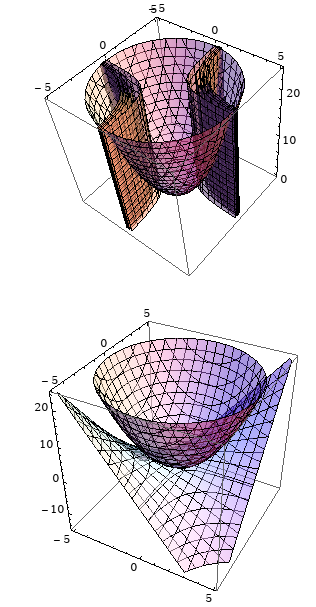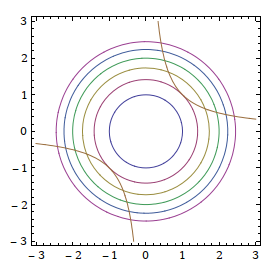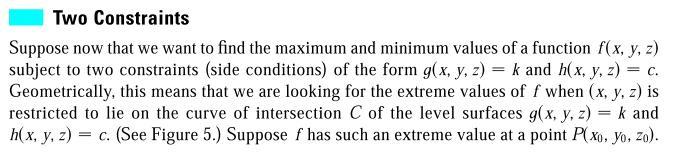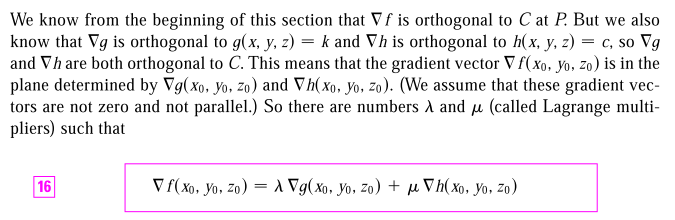## Lagrange multiplier: intuition and proof

Lagrange multiplier: intuition and proof

Lagrange multiplier is a technique of optimization under contraints. Take $f(x, y) = x^2 + y^2$ as an example, when there is no contraint, we just find the critical points by solving $\nabla f = 0$. What if we impose some contraint on it, i.e. we require that $xy = 1$?

Let's visualize the surfaces and curves first:Visualize $f(x, y) = x^2 + y^2$ and $xy = 1$ (mathematica code)
Click to toggle code (may have to try twice)
ContourPlot3D[{
x^2+y^2-z==0,
x y-1==0,
x y-1==1,
x y-1==1.5,
x y-1==2,
x y-1==2.5
},
{x, -5, 5}, {y, -5, 5},
{z, 0, 24},
ContourStyle -> Opacity@.5]

ContourPlot3D[{
x^2+y^2-z==0,
x y-z==0
},
{x, -5, 5}, {y, -5, 5},
{z, -15, 24},
ContourStyle -> Opacity@.5]

ContourPlot[{
x^2+y^2==1,
x^2+y^2==2,
x^2+y^2==3,
x^2+y^2==4,
x^2+y^2==5,
x^2+y^2==6,
x y==1
}, {x, -3, 3}, {y, -3, 3}]



We are dealing with three variables, $x, y, z$, and z is a function of x and y, so in this case, whenever we talk about gradient, we are talking about a 2-dimensional vector. The constraint $g = xy = 1$ is a curve, it is one dimensional, but in a 3D world, it's repeated through all levels of z, and turned into a "degenerate" surface. (fig. 1). Fig.3 is the most important, it captures the essence of Lagrange multiplier.

Imagine a point moving along the contraint curve, and as the $x-y$ coordinates vary, $f(x, y)$ changes accordingly, when $f$ reaches its extremum, its rate of change, at that moment, is 0. Suppose the directional vector at the point is $u$, then in the language of directional derivative, we have

\begin{align*} \nabla f \cdot u = 0 \end{align*}

But we also have $\nabla g \cdot u = 0$ since the curve $xy = 1$ is a level curve of $g$, therefore $\nabla f$ and $\nabla g$ are parallel, i.e. $$\nabla f = \lambda \nabla g$$.

$\lambda$ is called the Lagrange multiplier.

The idea can be generalized to more constraints:This generalization takes quite a bit imagination in the 3D world. The level curves turns into level surfaces, and the constraint level curve also turns into the constraint level surface.

When there is only one contratin surface, $g(x, y, z) = c$, imagine an arbitrary curve runing through the optimal point, then at that instant, the changing rate of $f$ is zero, let the directional vector at the optimal point be $u$, the in the language of directional derivatives, $\nabla f(x, y, z) \cdot u = 0$. Since $u$ is arbitrary, we know $\nabla f$ is perpendicular to the tangent plane at the optimal point, which also means $\nabla f = \lambda \nabla g$.

When there are two constraints $g, \; h$, we have three cases:

First, the two constraints have no points in common, then we don't have a solution at all, it's a hopeless case.

Second, they have only one point in common, i.e. they touch each other at that point. This is a trivial case.

Third, they intersect at a curve. Then $\nabla f$ is perpendicular to the curve at the optimal point. $\nabla g, \nabla h$ are also perpendicular to the curve there, so the curve is perpendicular to the plane determined by the gradients $\nabla f, \; \nabla h$, and $\nabla f$ must be in that plane.